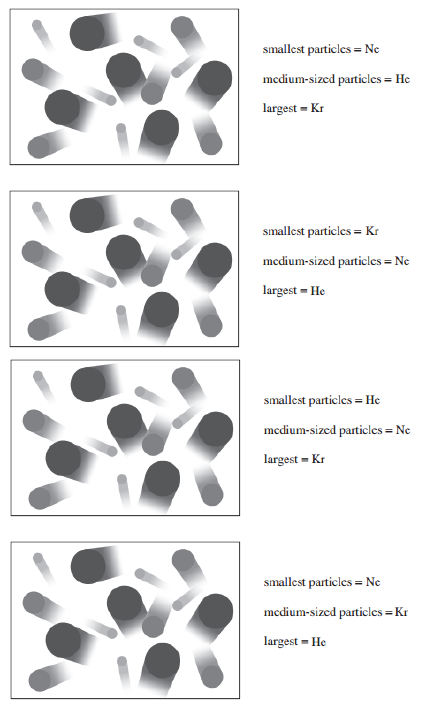# Problem: Select a depiction of a gas sample, as described by kinetic molecular theory, containing equal molar amounts of helium, neon, and krypton. Each atom has a "tail" to represent its velocity relative to the others in the mixture.

###### FREE Expert Solution

Recall that the molecular speed of a gas is given by:

where R = gas constant, T = temperature, and M = molar mass of the gas.

99% (254 ratings)###### Problem Details

Select a depiction of a gas sample, as described by kinetic molecular theory, containing equal molar amounts of helium, neon, and krypton. Each atom has a "tail" to represent its velocity relative to the others in the mixture.Frequently Asked Questions

What scientific concept do you need to know in order to solve this problem?

Our tutors have indicated that to solve this problem you will need to apply the Kinetic Molecular Theory concept. You can view video lessons to learn Kinetic Molecular Theory. Or if you need more Kinetic Molecular Theory practice, you can also practice Kinetic Molecular Theory practice problems.

What professor is this problem relevant for?

Based on our data, we think this problem is relevant for Professor Smith's class at SCAR.